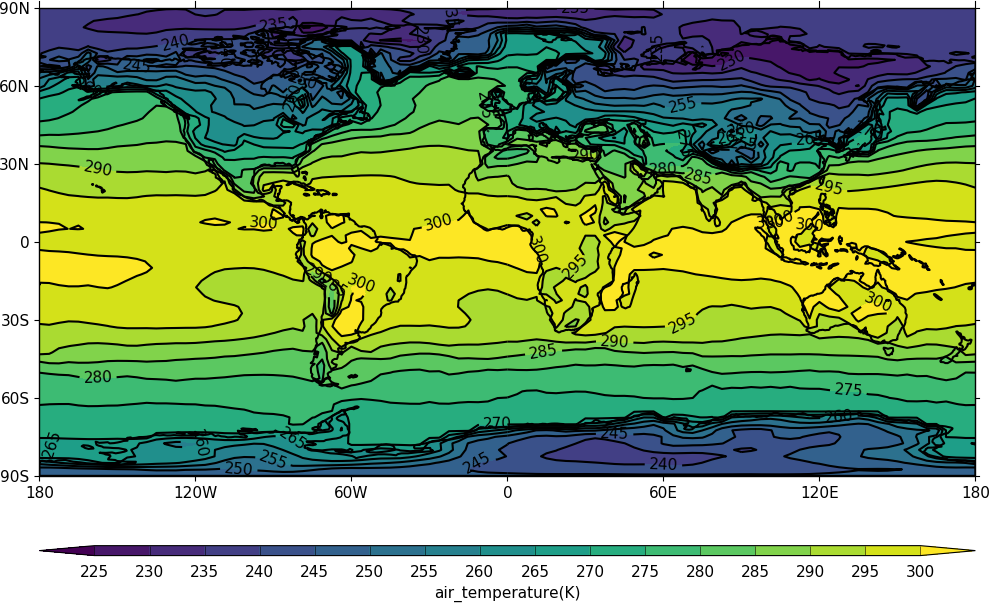# cf-plot homepage¶

cf-plot is a set of Python routines for making the common contour, vector and line plots that climate researchers use. The data to make a contour plot can be passed to cf-plot using cf-python as in the following example.```import cf
import cfplot as cfp